Скачать презентацию Chapter 6 The mole Measuring Matter What

bdbcf622f06ab26c9b7d3f85cbcddf62.ppt

• Количество слайдов: 47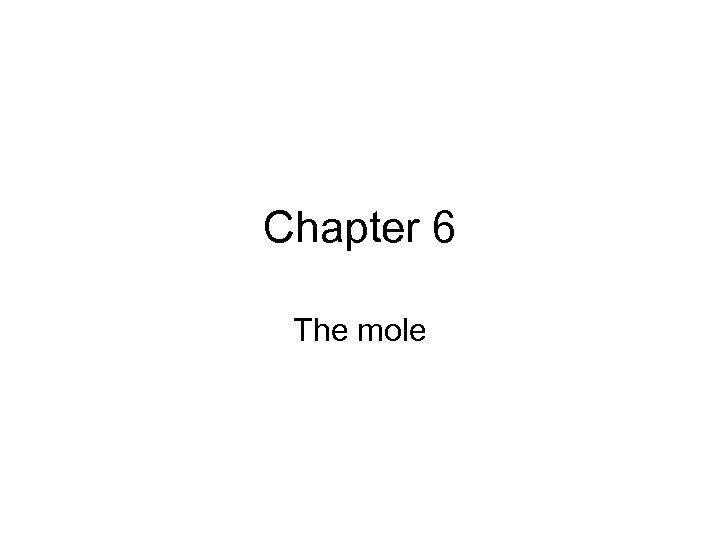Chapter 6 The mole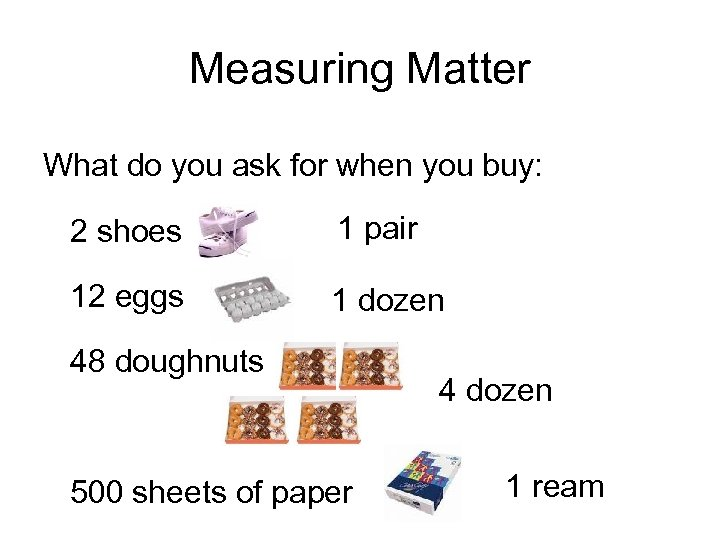Measuring Matter What do you ask for when you buy: 2 shoes 1 pair 12 eggs 1 dozen 48 doughnuts 500 sheets of paper 4 dozen 1 ream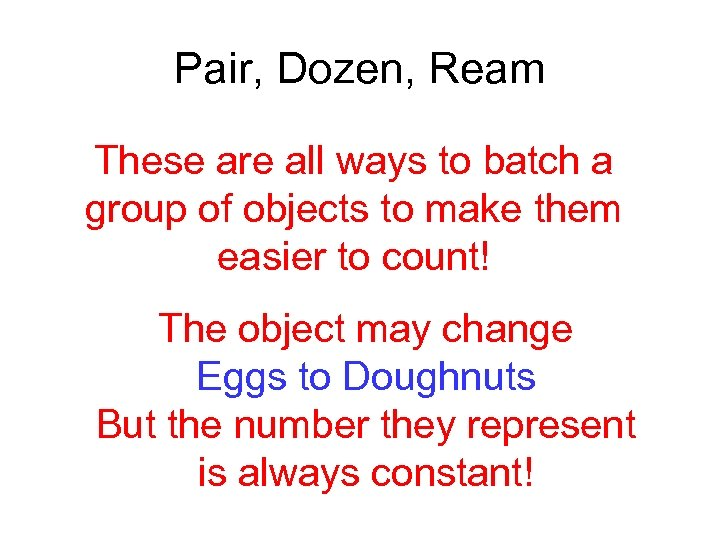Pair, Dozen, Ream These are all ways to batch a group of objects to make them easier to count! The object may change Eggs to Doughnuts But the number they represent is always constant!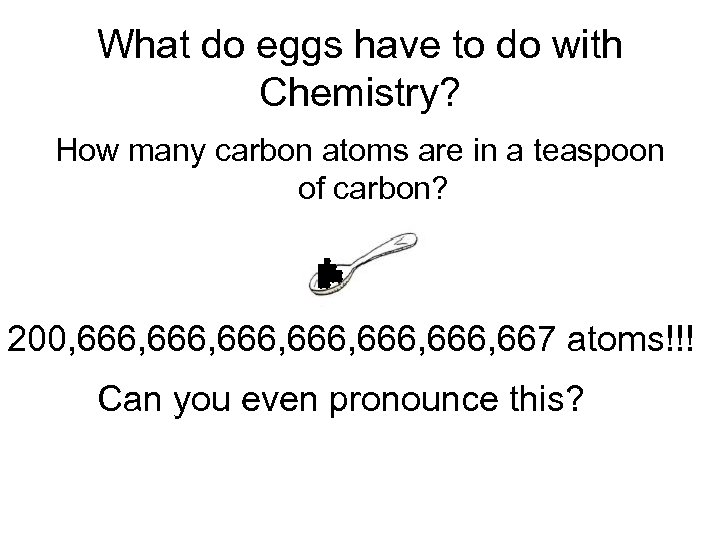What do eggs have to do with Chemistry? How many carbon atoms are in a teaspoon of carbon? 200, 666, 666, 667 atoms!!! Can you even pronounce this?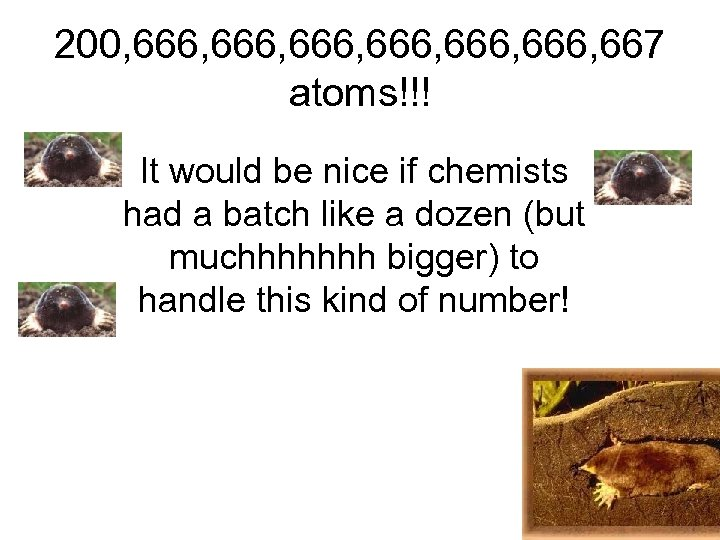200, 666, 666, 667 atoms!!! It would be nice if chemists had a batch like a dozen (but muchhhhhhh bigger) to handle this kind of number!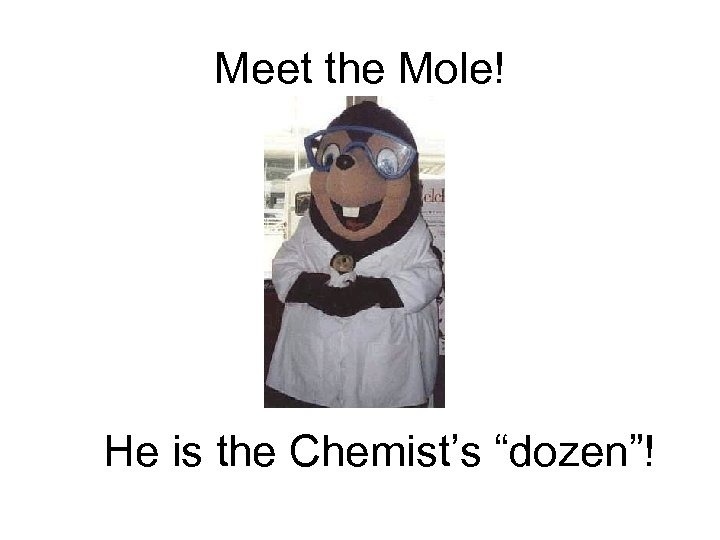Meet the Mole! He is the Chemist’s “dozen”!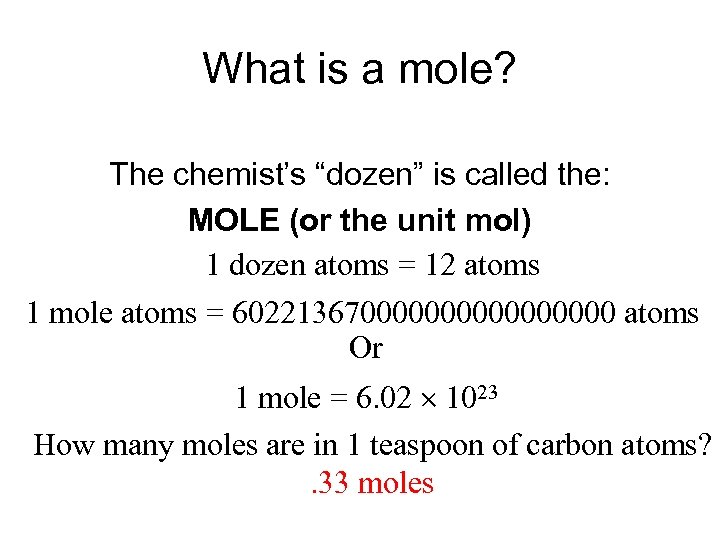What is a mole? The chemist’s “dozen” is called the: MOLE (or the unit mol) 1 dozen atoms = 12 atoms 1 mole atoms = 6022136700000000 atoms Or 1 mole = 6. 02 1023 How many moles are in 1 teaspoon of carbon atoms? . 33 moles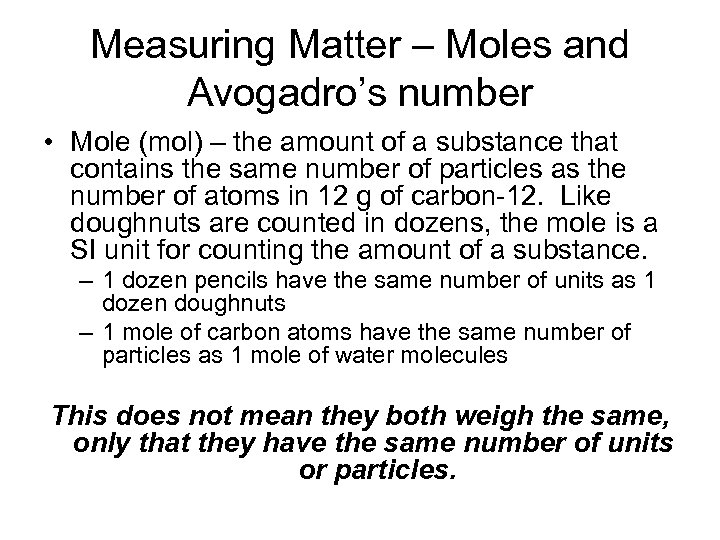Measuring Matter – Moles and Avogadro’s number • Mole (mol) – the amount of a substance that contains the same number of particles as the number of atoms in 12 g of carbon-12. Like doughnuts are counted in dozens, the mole is a SI unit for counting the amount of a substance. – 1 dozen pencils have the same number of units as 1 dozen doughnuts – 1 mole of carbon atoms have the same number of particles as 1 mole of water molecules This does not mean they both weigh the same, only that they have the same number of units or particles.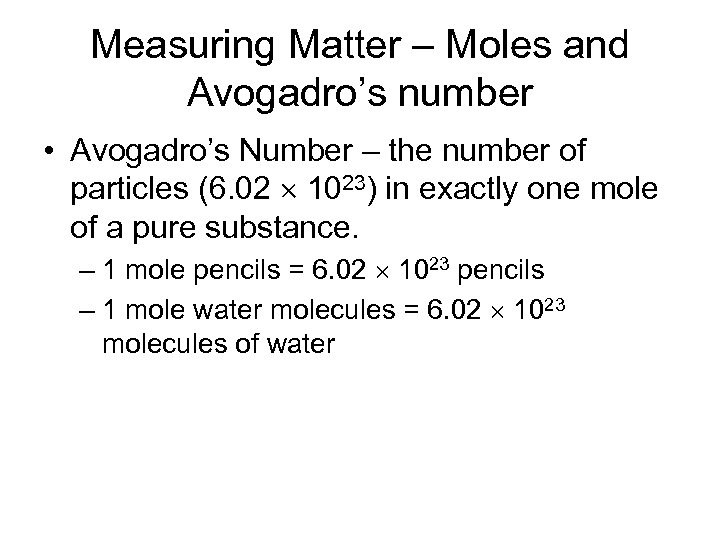Measuring Matter – Moles and Avogadro’s number • Avogadro’s Number – the number of particles (6. 02 1023) in exactly one mole of a pure substance. – 1 mole pencils = 6. 02 1023 pencils – 1 mole water molecules = 6. 02 1023 molecules of water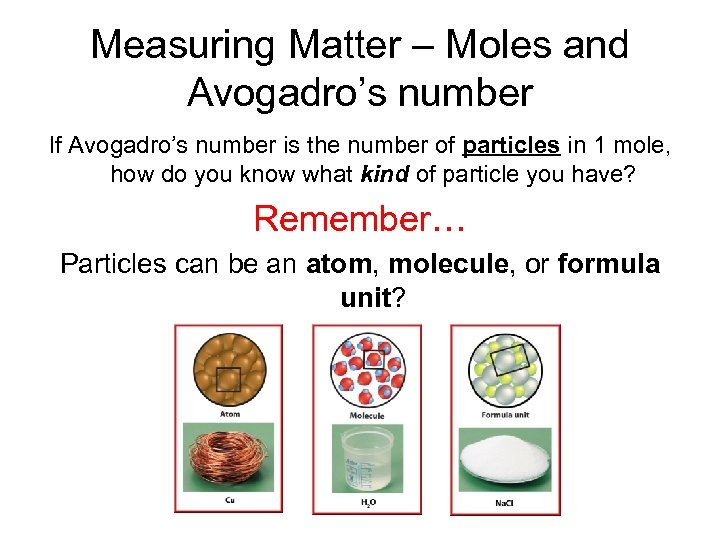Measuring Matter – Moles and Avogadro’s number If Avogadro’s number is the number of particles in 1 mole, how do you know what kind of particle you have? Remember… Particles can be an atom, molecule, or formula unit?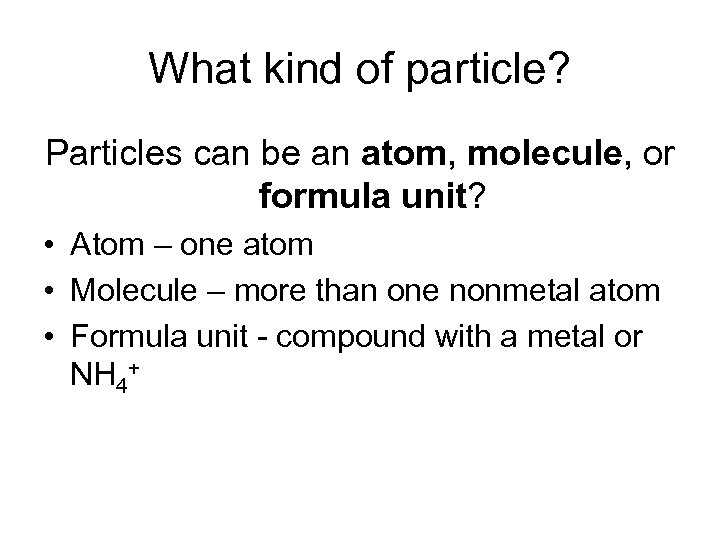What kind of particle? Particles can be an atom, molecule, or formula unit? • Atom – one atom • Molecule – more than one nonmetal atom • Formula unit - compound with a metal or NH 4+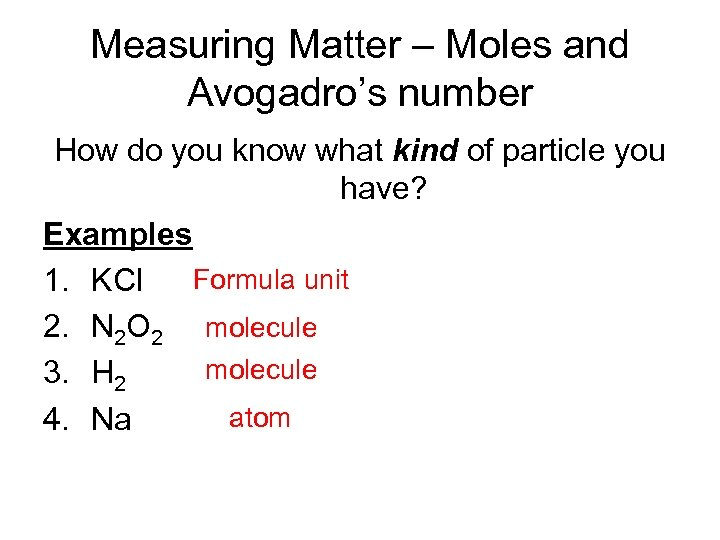Measuring Matter – Moles and Avogadro’s number How do you know what kind of particle you have? Examples Formula unit 1. KCl 2. N 2 O 2 molecule 3. H 2 atom 4. Na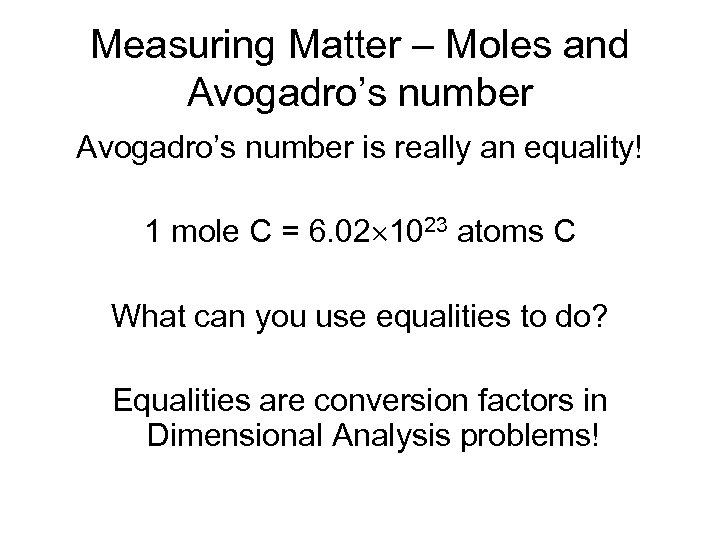Measuring Matter – Moles and Avogadro’s number is really an equality! 1 mole C = 6. 02 1023 atoms C What can you use equalities to do? Equalities are conversion factors in Dimensional Analysis problems!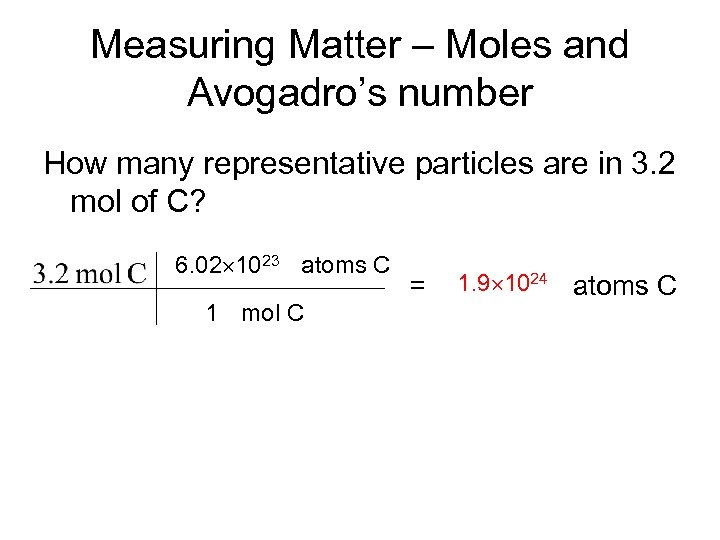Measuring Matter – Moles and Avogadro’s number How many representative particles are in 3. 2 mol of C? 6. 02 1023 atoms C 1 mol C = 1. 9 1024 atoms C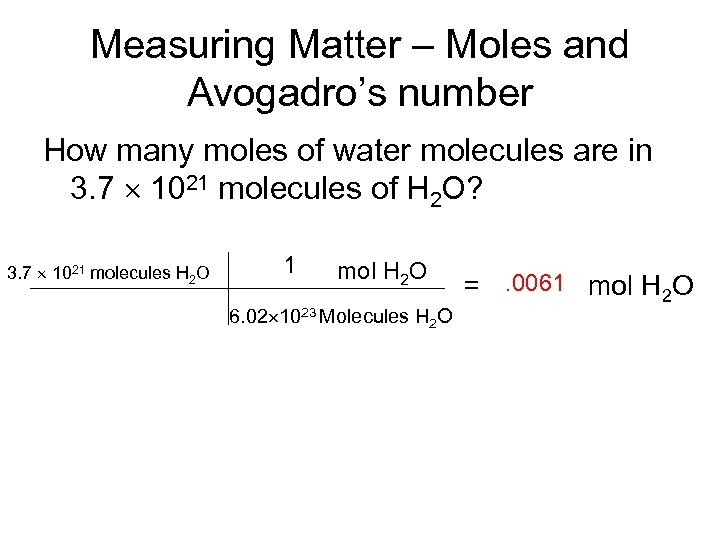Measuring Matter – Moles and Avogadro’s number How many moles of water molecules are in 3. 7 1021 molecules of H 2 O? 3. 7 1021 molecules H 2 O 1 mol H 2 O 6. 02 1023 Molecules H 2 O =. 0061 mol H 2 O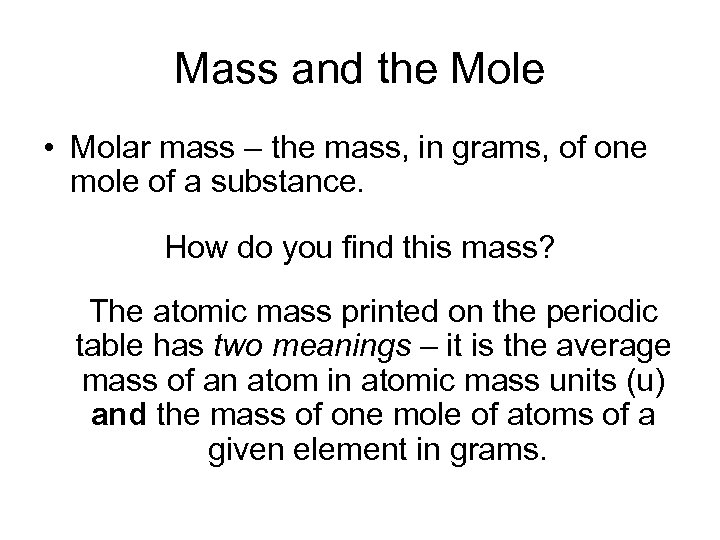Mass and the Mole • Molar mass – the mass, in grams, of one mole of a substance. How do you find this mass? The atomic mass printed on the periodic table has two meanings – it is the average mass of an atom in atomic mass units (u) and the mass of one mole of atoms of a given element in grams.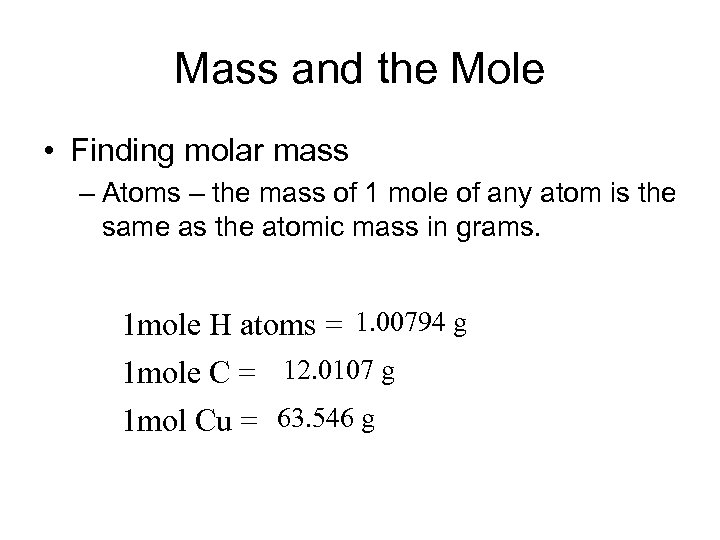Mass and the Mole • Finding molar mass – Atoms – the mass of 1 mole of any atom is the same as the atomic mass in grams. 1 mole H atoms = 1. 00794 g 1 mole C = 12. 0107 g 1 mol Cu = 63. 546 g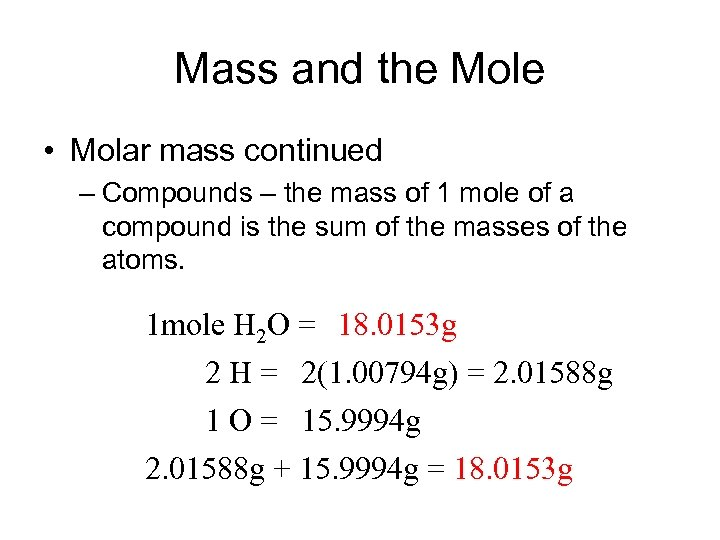Mass and the Mole • Molar mass continued – Compounds – the mass of 1 mole of a compound is the sum of the masses of the atoms. 1 mole H 2 O = 18. 0153 g 2 H = 2(1. 00794 g) = 2. 01588 g 1 O = 15. 9994 g 2. 01588 g + 15. 9994 g = 18. 0153 g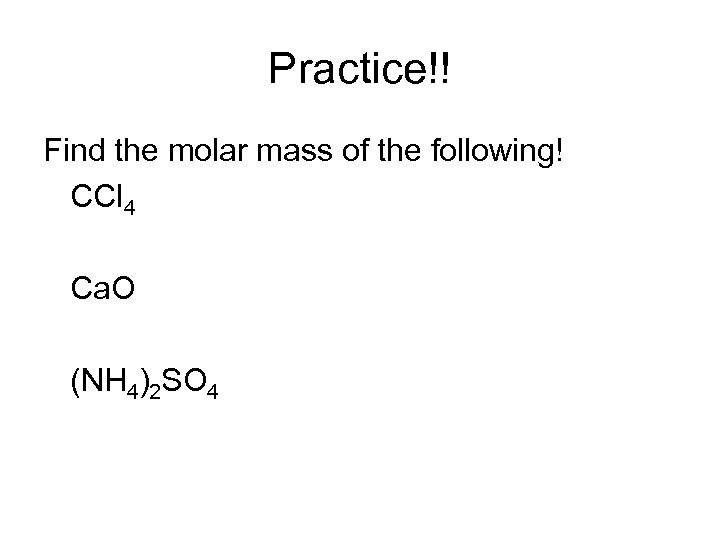Practice!! Find the molar mass of the following! CCl 4 Ca. O (NH 4)2 SO 4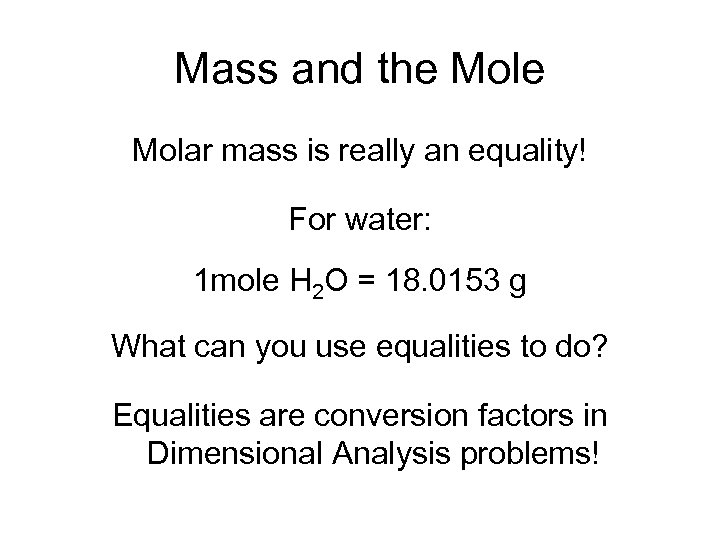Mass and the Molar mass is really an equality! For water: 1 mole H 2 O = 18. 0153 g What can you use equalities to do? Equalities are conversion factors in Dimensional Analysis problems!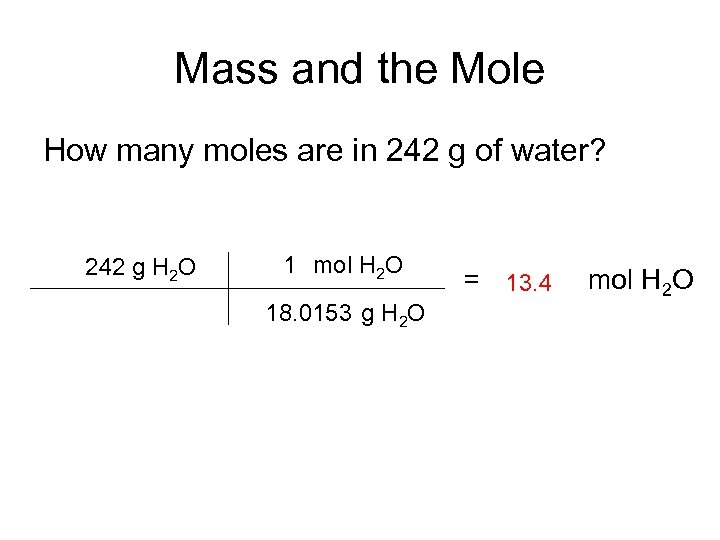Mass and the Mole How many moles are in 242 g of water? 242 g H 2 O 1 mol H 2 O 18. 0153 g H 2 O = 13. 4 mol H 2 O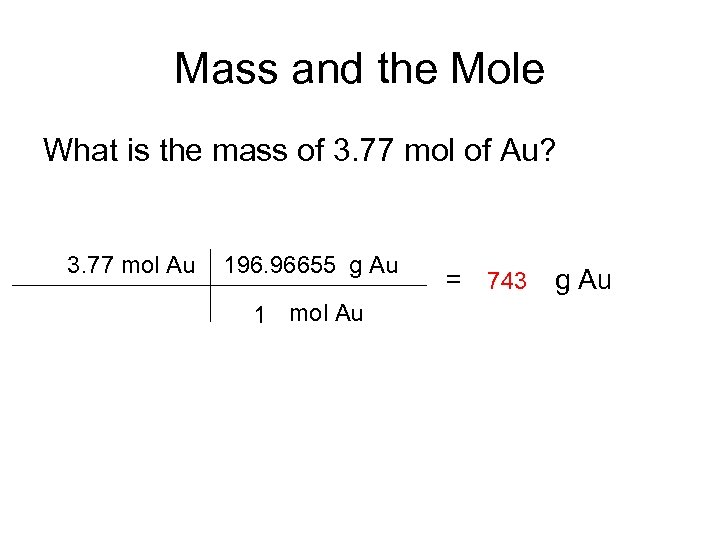Mass and the Mole What is the mass of 3. 77 mol of Au? 3. 77 mol Au 196. 96655 g Au 1 mol Au = 743 g Au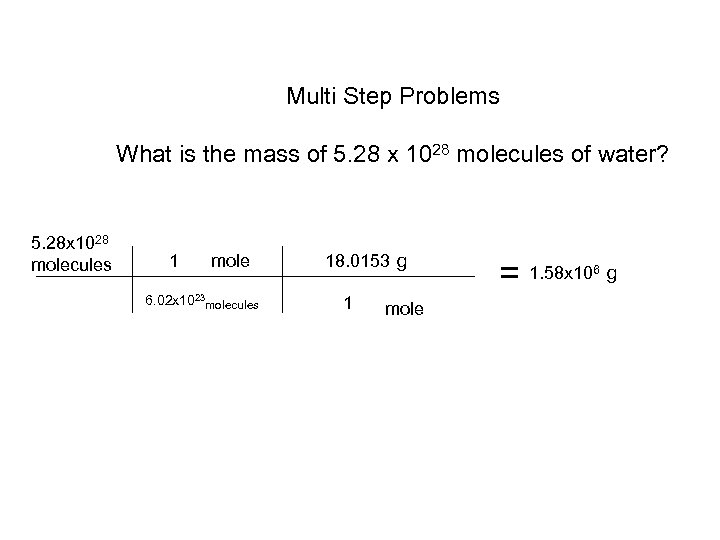Multi Step Problems What is the mass of 5. 28 x 1028 molecules of water? 5. 28 x 1028 molecules 1 mole 6. 02 x 1023 molecules 18. 0153 g 1 mole = 1. 58 x 10 6 g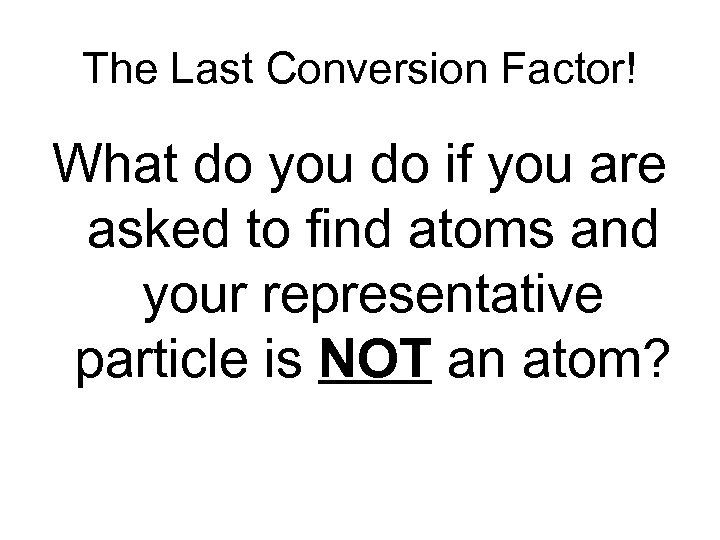The Last Conversion Factor! What do you do if you are asked to find atoms and your representative particle is NOT an atom?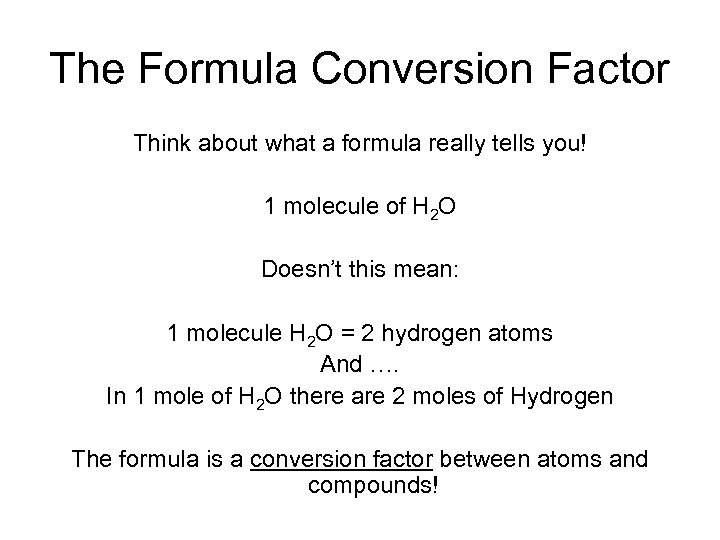The Formula Conversion Factor Think about what a formula really tells you! 1 molecule of H 2 O Doesn’t this mean: 1 molecule H 2 O = 2 hydrogen atoms And …. In 1 mole of H 2 O there are 2 moles of Hydrogen The formula is a conversion factor between atoms and compounds!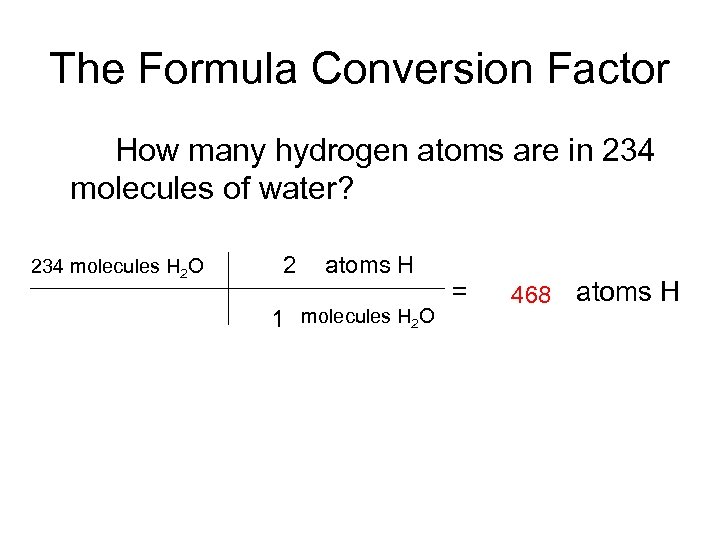The Formula Conversion Factor How many hydrogen atoms are in 234 molecules of water? 234 molecules H 2 O 2 atoms H 1 molecules H 2 O = 468 atoms H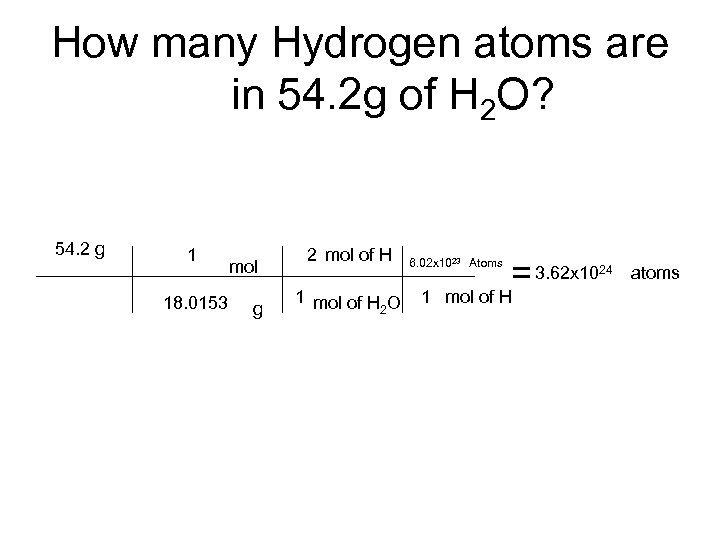How many Hydrogen atoms are in 54. 2 g of H 2 O? 54. 2 g 1 18. 0153 mol g 2 mol of H 1 mol of H O 2 6. 02 x 1023 Atoms = 3. 62 x 10 1 mol of H 24 atoms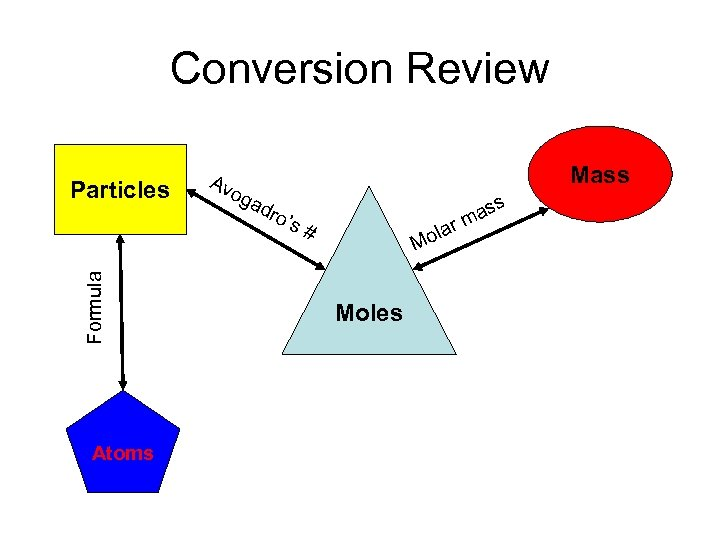Conversion Review Formula Particles Atoms Av og Mass ad ro’ s# la ass rm Mo Moles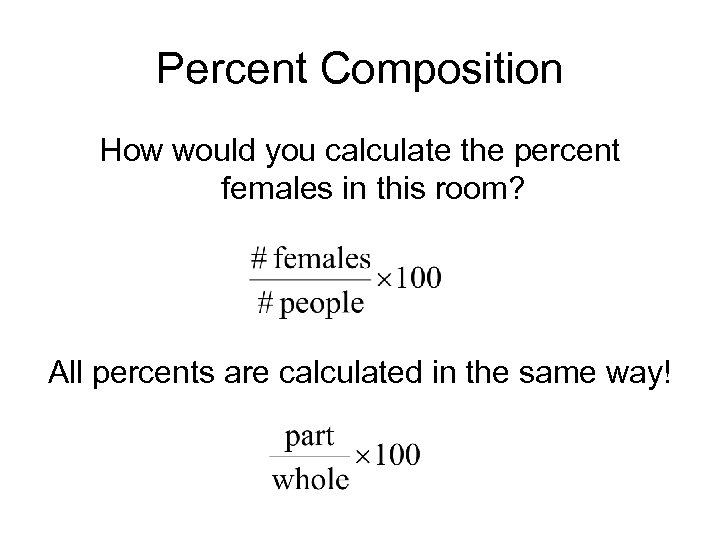Percent Composition How would you calculate the percent females in this room? All percents are calculated in the same way!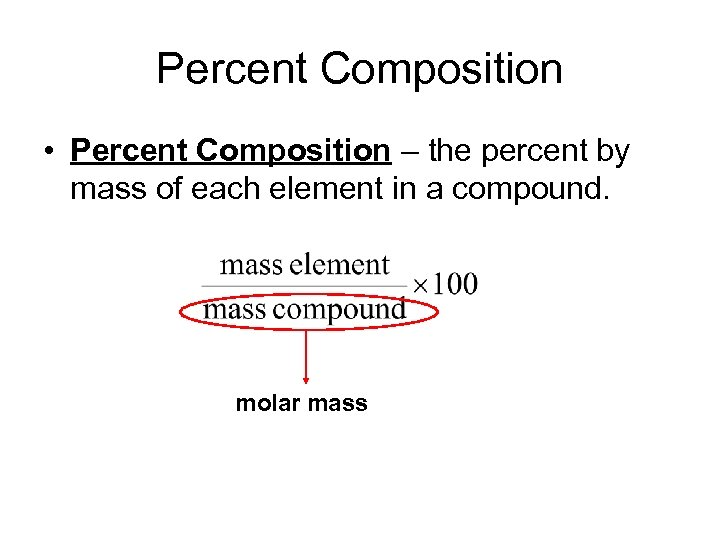Percent Composition • Percent Composition – the percent by mass of each element in a compound. molar mass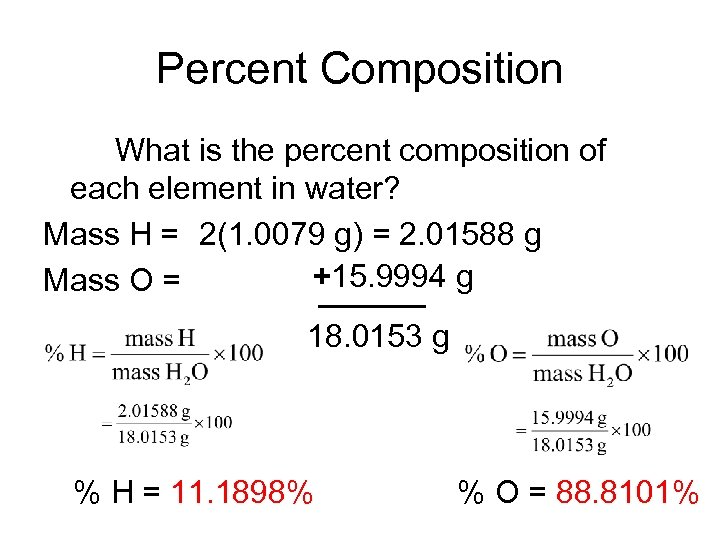Percent Composition What is the percent composition of each element in water? Mass H = 2(1. 0079 g) = 2. 01588 g +15. 9994 g Mass O = 18. 0153 g % H = 11. 1898% % O = 88. 8101%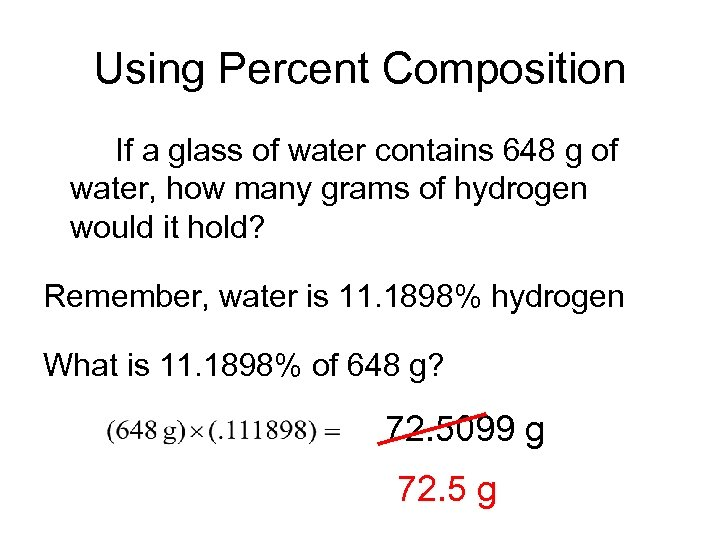Using Percent Composition If a glass of water contains 648 g of water, how many grams of hydrogen would it hold? Remember, water is 11. 1898% hydrogen What is 11. 1898% of 648 g? 72. 5099 g 72. 5 g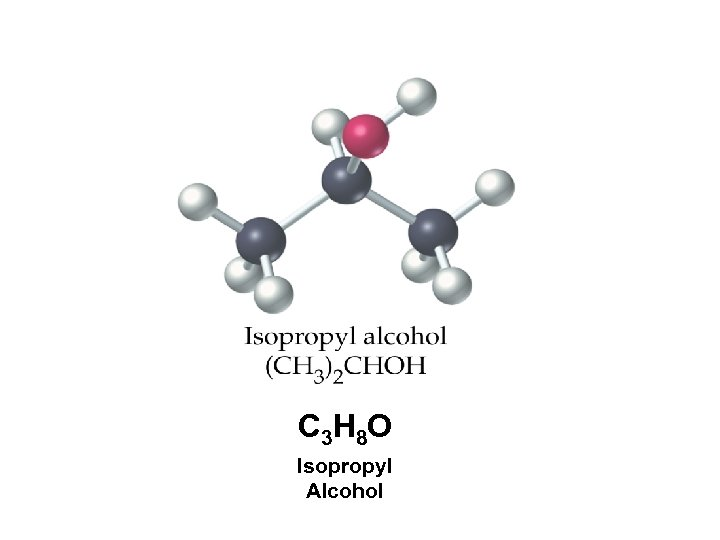C 3 H 8 O Isopropyl Alcohol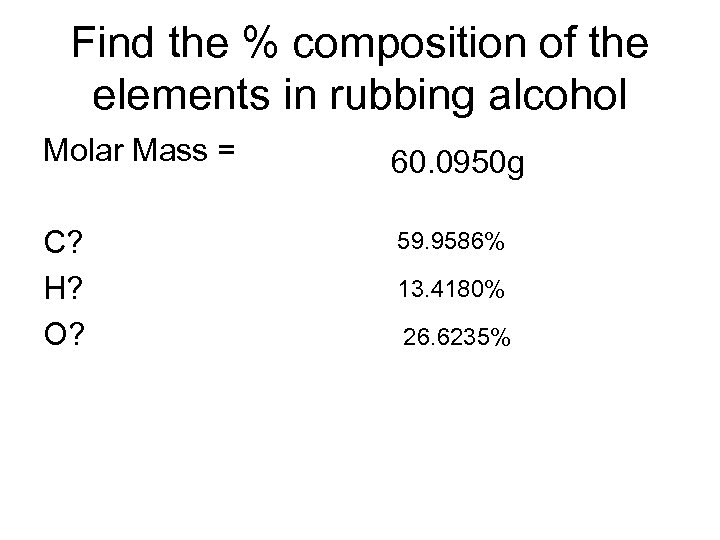Find the % composition of the elements in rubbing alcohol Molar Mass = 60. 0950 g C? H? O? 59. 9586% 13. 4180% 26. 6235%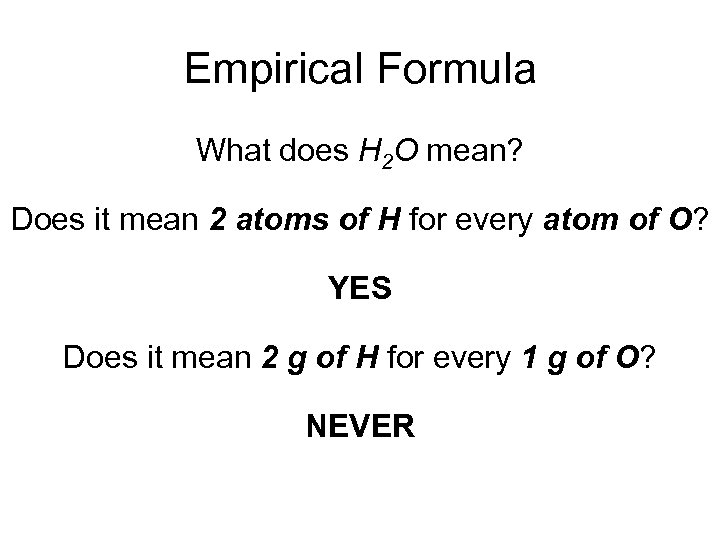Empirical Formula What does H 2 O mean? Does it mean 2 atoms of H for every atom of O? YES Does it mean 2 g of H for every 1 g of O? NEVER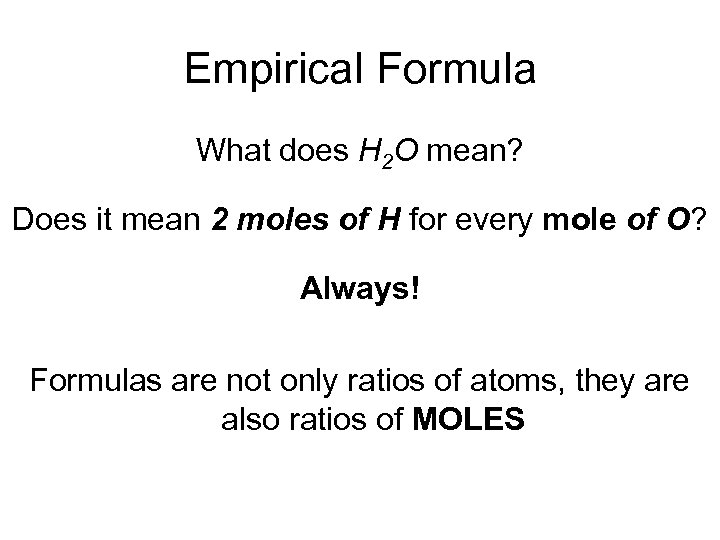Empirical Formula What does H 2 O mean? Does it mean 2 moles of H for every mole of O? Always! Formulas are not only ratios of atoms, they are also ratios of MOLES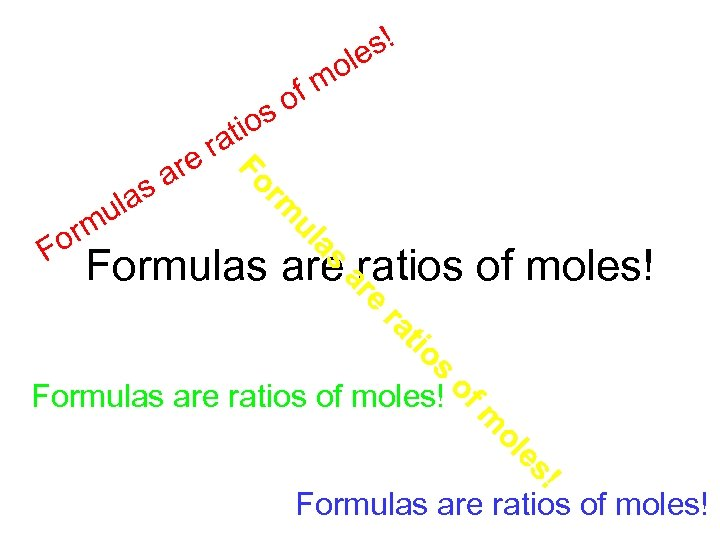a ul or F o m rm Fo ula m are s tio ra of s s! le s Formulas are ratios of moles! e ar s tio ra ! es ol m of Formulas are ratios of moles!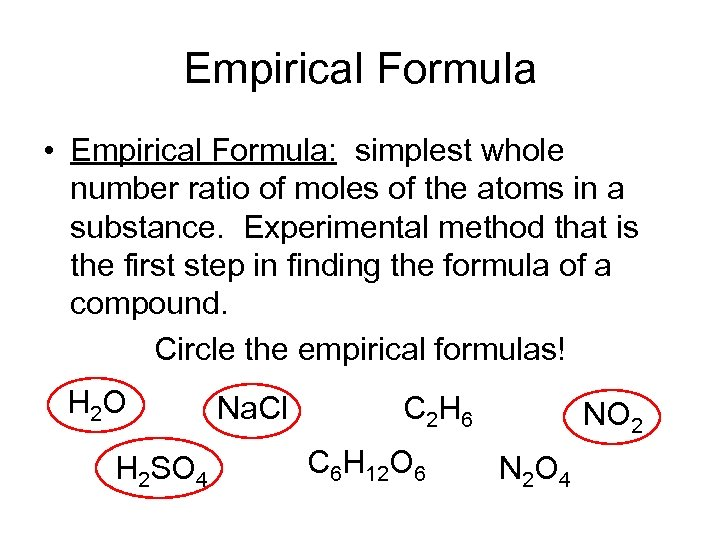Empirical Formula • Empirical Formula: simplest whole number ratio of moles of the atoms in a substance. Experimental method that is the first step in finding the formula of a compound. Circle the empirical formulas! H 2 O H 2 SO 4 Na. Cl C 2 H 6 C 6 H 12 O 6 NO 2 N 2 O 4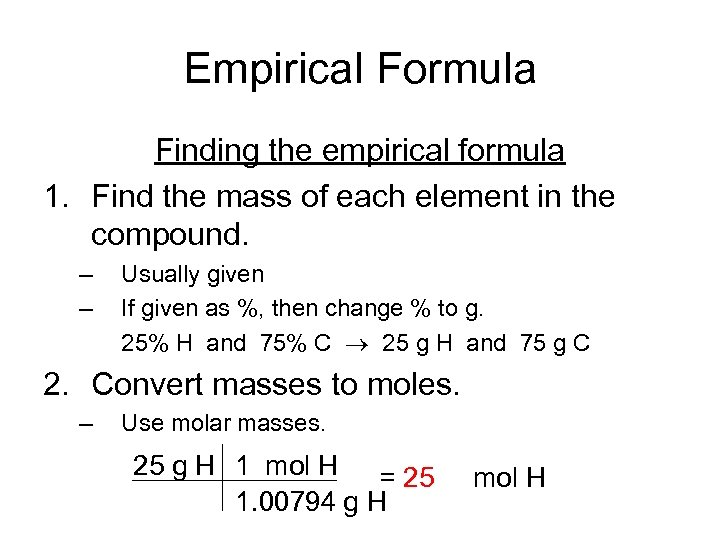Empirical Formula Finding the empirical formula 1. Find the mass of each element in the compound. – – Usually given If given as %, then change % to g. 25% H and 75% C 25 g H and 75 g C 2. Convert masses to moles. – Use molar masses. 25 g H 1 mol H = 25 1. 00794 g H mol H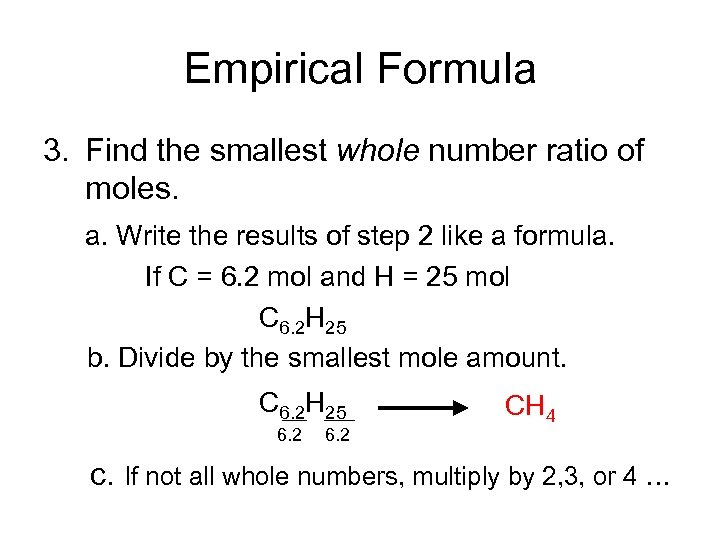Empirical Formula 3. Find the smallest whole number ratio of moles. a. Write the results of step 2 like a formula. If C = 6. 2 mol and H = 25 mol C 6. 2 H 25 b. Divide by the smallest mole amount. C 6. 2 H 25 6. 2 CH 4 c. If not all whole numbers, multiply by 2, 3, or 4 …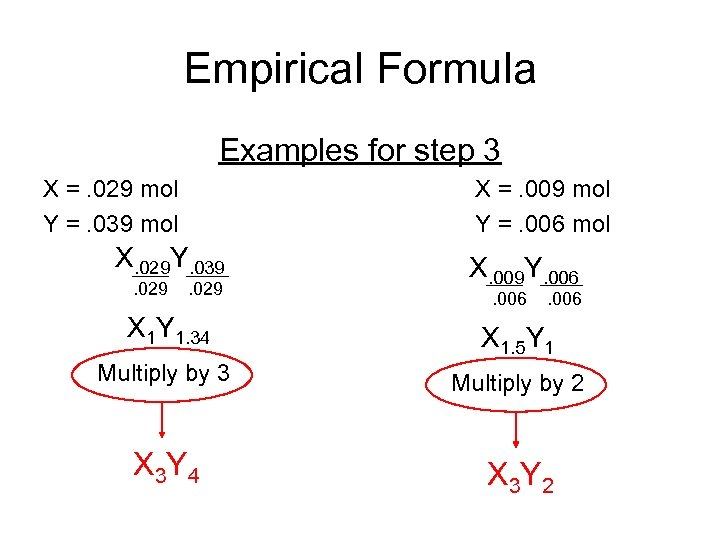Empirical Formula Examples for step 3 X =. 029 mol Y =. 039 mol X =. 009 mol Y =. 006 mol X. 029 Y. 039. 029 X. 009 Y. 006 X 1 Y 1. 34 X 1. 5 Y 1 Multiply by 3 Multiply by 2 X 3 Y 4 X 3 Y 2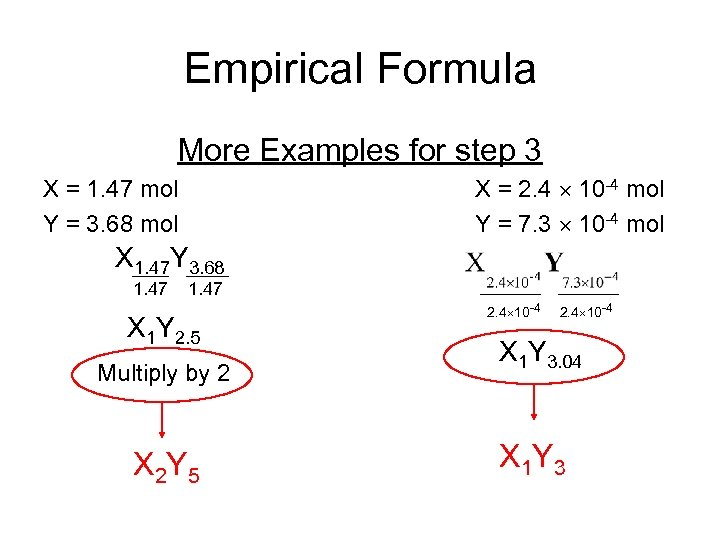Empirical Formula More Examples for step 3 X = 2. 4 10 -4 mol Y = 7. 3 10 -4 mol X = 1. 47 mol Y = 3. 68 mol X 1. 47 Y 3. 68 1. 47 X 1 Y 2. 5 Multiply by 2 X 2 Y 5 2. 4 10 -4 X 1 Y 3. 04 X 1 Y 3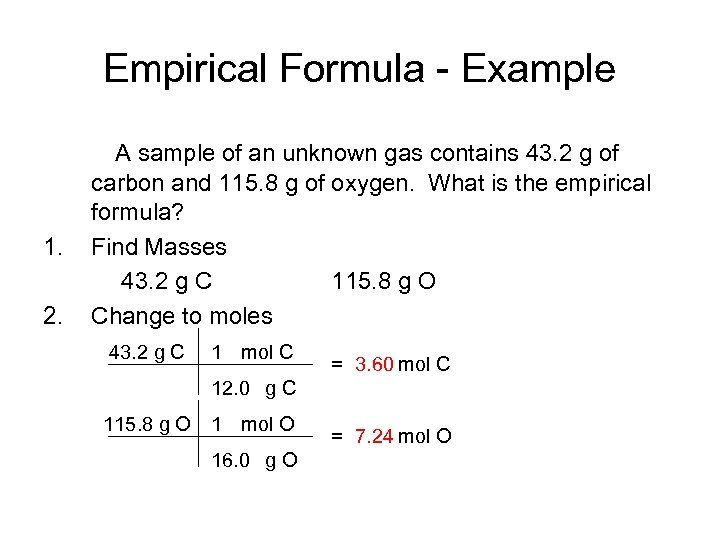Empirical Formula - Example 1. 2. A sample of an unknown gas contains 43. 2 g of carbon and 115. 8 g of oxygen. What is the empirical formula? Find Masses 43. 2 g C 115. 8 g O Change to moles 43. 2 g C 1 mol C = 3. 60 mol C 12. 0 g C 115. 8 g O 1 mol O 16. 0 g O = 7. 24 mol O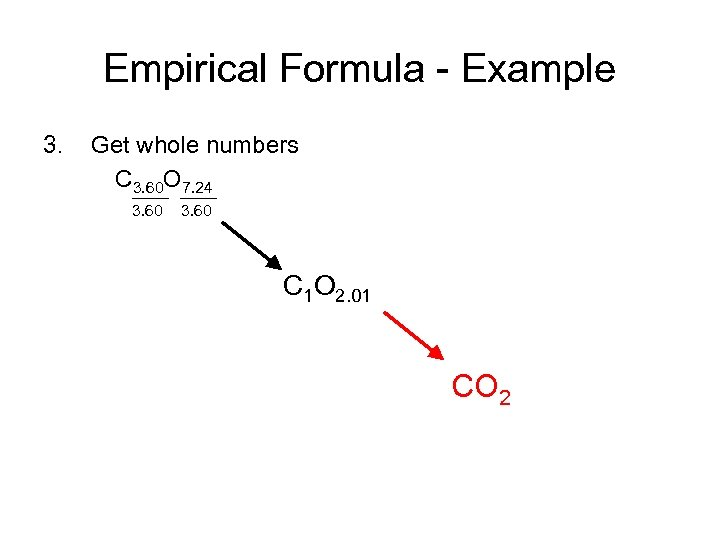Empirical Formula - Example 3. Get whole numbers C 3. 60 O 7. 24 3. 60 C 1 O 2. 01 CO 2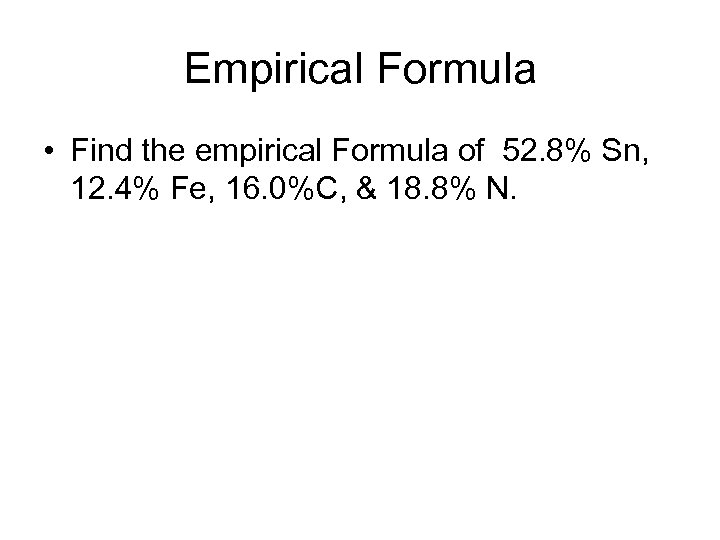Empirical Formula • Find the empirical Formula of 52. 8% Sn, 12. 4% Fe, 16. 0%C, & 18. 8% N.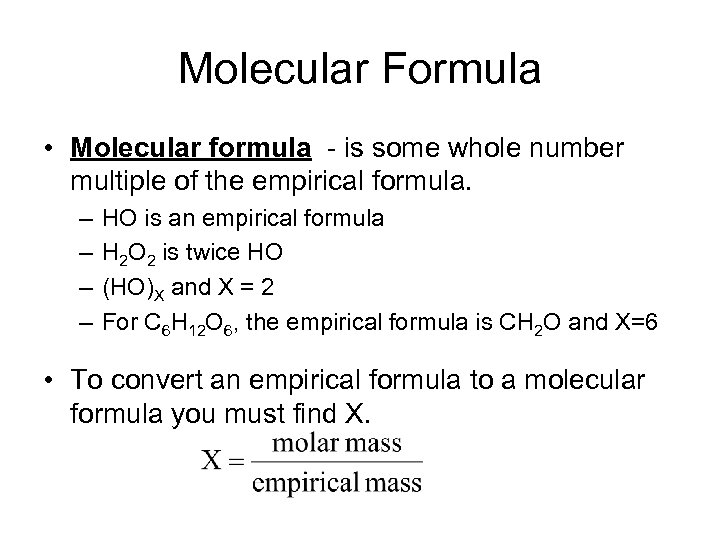Molecular Formula • Molecular formula - is some whole number multiple of the empirical formula. – – HO is an empirical formula H 2 O 2 is twice HO (HO)X and X = 2 For C 6 H 12 O 6, the empirical formula is CH 2 O and X=6 • To convert an empirical formula to a molecular formula you must find X.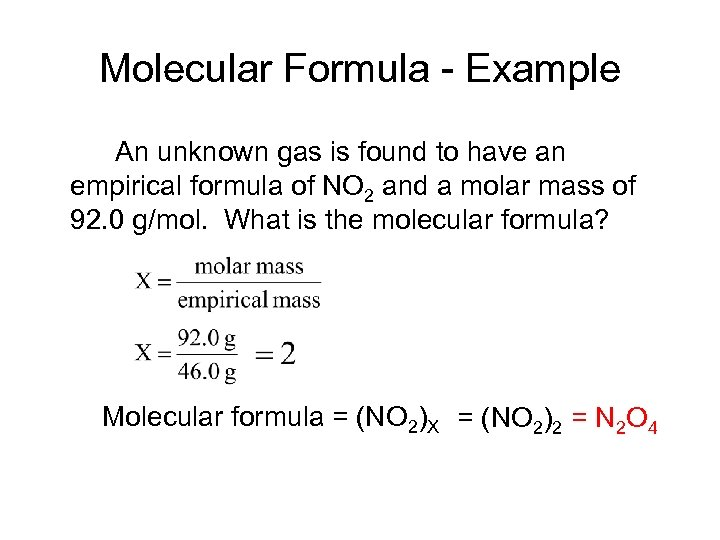Molecular Formula - Example An unknown gas is found to have an empirical formula of NO 2 and a molar mass of 92. 0 g/mol. What is the molecular formula? Molecular formula = (NO 2)X = (NO 2)2 = N 2 O 4